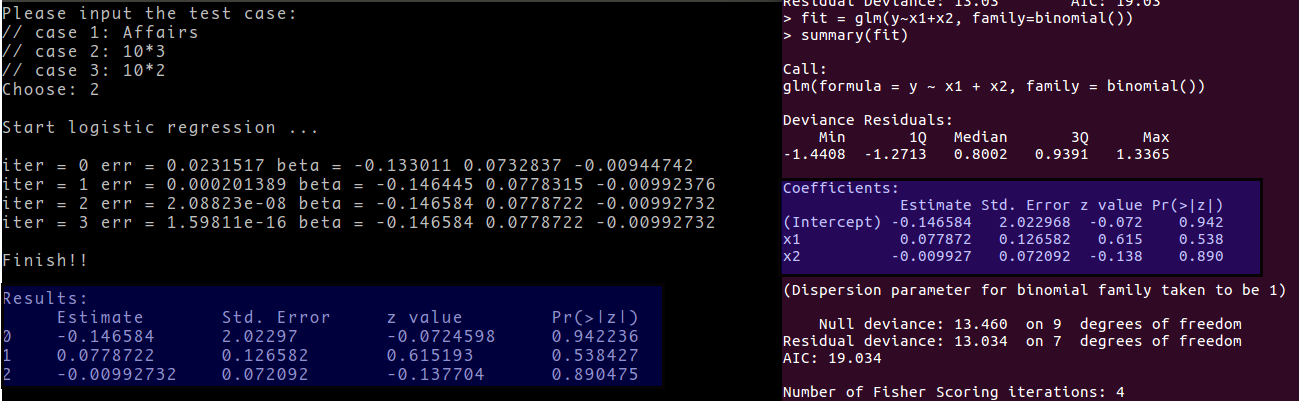# Estimate Parameters in Logistic Regression

##### Posted on Jul 30, 20170 Comments
Tags: Logistic Regression, MLE

## Background

Assuming $\boldsymbol y$ is a $n\times 1$ response variable, and $y_i\sim B(1, \pi_i)$. $\boldsymbol x_1,\ldots,\boldsymbol x_p$ are $p$ explanatory variables.

Then the likelihood of $\boldsymbol y$ is

$L(\pi_1,\ldots,\pi_n)=\prod\limits_{i=1}^n\pi_i^{y_i}(1-\pi_i)^{1-y_i}$

and the log-likelihood is

$l(\pi_1,\ldots,\pi_n)=\sum\limits_{i=1}^n[y_i\log\pi_i+(1-y_i)\log(1-\pi_i))]$

The logistic regression said that

$y_i=\logit(\pi_i) = \log\frac{\pi_i}{1-\pi_i}=\boldsymbol x_i'\boldsymbol\beta$

where

$\boldsymbol\beta = (\beta_0,\beta_1,\ldots,\beta_p)\qquad \boldsymbol x_i = (1, x_{i1}, \ldots, x_{ip})'$

then we have

$\pi_i = \frac{\exp(\boldsymbol x_i'\boldsymbol\beta)}{1+\exp(\boldsymbol x_i'\boldsymbol\beta)}$

Thus, the log-likelihood could be

$l(\boldsymbol{\beta})=\sum\limits_{i=1}^n[y_i\boldsymbol {x_i'}\boldsymbol{\beta}-\log(1+\exp(\boldsymbol {x_i'}\boldsymbol{\beta}))]$

## Maximum Likelihood Estimate

We need to find $\boldsymbol \beta$ to minimize $l(\boldsymbol \beta)$, which means that

$\frac{\partial l(\boldsymbol \beta)}{\partial \boldsymbol \beta}=\sum\limits_{i=1}^n(y_i-\frac{\exp(\boldsymbol {x_i'}\boldsymbol{\beta})}{1+\exp(\boldsymbol x_i'\boldsymbol\beta)})\boldsymbol x_i = 0$

We adopt Newton-Raphson Algorithm (Multivariate version) to solve $\boldsymbol \beta$ numerically.

Let

$f_j(\boldsymbol \beta) = \sum\limits_{i=1}^n(y_i-\frac{\exp(\boldsymbol x_i'\boldsymbol\beta)}{1+\exp(\boldsymbol x_i'\boldsymbol\beta)})\boldsymbol x_{ij} = 0$

then

$\frac{\partial l(\boldsymbol \beta)}{\partial \boldsymbol \beta} = \boldsymbol f=(f_0,\ldots, f_p)$

so

$\boldsymbol J = \left[ \begin{array}{cccc} \frac{\partial f_0}{\partial \beta_0} & \frac{\partial f_0}{\partial \beta_1} & \cdots & \frac{\partial f_0}{\partial \beta_p}\\ \frac{\partial f_1}{\partial \beta_0} & \frac{\partial f_1}{\partial \beta_1} & \cdots & \frac{\partial f_1}{\partial \beta_p}\\ \vdots & \vdots & \ddots & \vdots\\ \frac{\partial f_p}{\partial \beta_0} & \frac{\partial f_p}{\partial \beta_1} & \cdots & \frac{\partial f_p}{\partial \beta_p}\\ \end{array} \right]$

where

$\frac{\partial f_j}{\partial \beta_k} = -\sum\limits_{i=1}^n(1-\pi_i)\pi_ix_{ij}x_{ik}$

The Newton-Raphson formula for multi-variate problem is

$\boldsymbol \beta = \boldsymbol \beta - J^{-1}(\boldsymbol \beta)\boldsymbol f(\boldsymbol \beta)$

## Wald Test for Parameters

In the univariate case, the Wald statistic is

$\frac{(\hat \theta-\theta_0)}{var(\hat\theta)}$

which is compared against a chi-squared distribution.

Alternatively, the difference can be compared to a normal distribution. In this case, the test statistic is

$\frac{\hat\theta-\theta_0}{se(\hat\theta)}$

where $se(\hat\theta)$ is the standard error of the maximum likelihood estimate. Assuming $\mathbf H$ is the Hessian of the log-likelihood function $l$, then the vector $\sqrt{diag((-\mathbf H)^{-1})}$ is the estimate of the standard error of each parameter value at its maximum.

## Implement in C++ and R

Implement above algorithm and apply to an example, and compare the logistic regression results with glm(..., family = binomial()) in R.If you want to know more details, you can visit my github repository gsl_lm/logit.